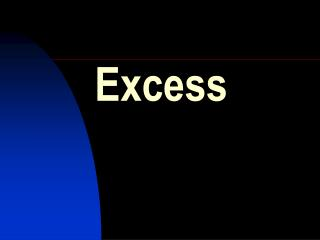DownloadDownload PresentationExcess

# Excess

Download Presentation## Excess

- - - - - - - - - - - - - - - - - - - - - - - - - - - E N D - - - - - - - - - - - - - - - - - - - - - - - - - - -
##### Presentation Transcript

1. Excess

2. mass n gfm n C V (l) Calculate the number of moles of each reactant:- Number of moles in 10g of CaCO3 = 10/100 = 0.1 Number of moles of HCl = 1 x 100/1000 = 0.1 HIGHER GRADE CHEMISTRY CALCULATIONS As soon as one of the reactants in a chemical reaction is used up the reaction stops. Any other reactant which is left over is said to be ‘in excess’. The reactant which is used up determines the mass of product formed. Calculation of involving excess Worked example. Which reactant is in excess when 10g of calcium carbonate reacts with 100cm3 of 1 mol l-1 hydrochloric acid? Write the balanced equation for the reaction and show mole ratio:- CaCO3 + 2HCl  CaCl2 + CO2 + H2O 1 mol 2 mol From equation 0.1 mol of CaCO3 needs 0.2 mol of HCl and as we only have 0.1 mol of HCl the CaCO3 is in excess.

3. Calculation of excess • Calculations for you to try. • What mass of calcium oxide is formed when 0.4 g of calcium reacts with 0.05 mole of oxygen? • 2Ca + O2 2CaO 2Ca + O2 2CaO 2 mol 1 mol Number of moles of Ca in 0.4 g = 0.4/40 = 0.01 From equation 2 mol of Ca reacts with 1 mol of O2. So 0.01 mol of Ca reacts with 0.005 mol of O2. As we have 0.05 mol of O2 it is in excess. All 0.01 mol of Ca is used up From equation 0.01 mol of Ca will produce 0.01 mol of CaO 0.01 mol of CaO weighs 0.01 x 56 = 0.56 g Higher Grade Chemistry

4. Calculation of excess • Calculations for you to try. • What mass of hydrogen is formed when 3.27g of zinc is reacted with 25cm3 of 2 mol l-1 hydrochloric acid? • Zn + 2HCl  ZnCl2 + H2 Zn + 2HCl  ZnCl2 + H2 1 mol 2 mol Number of moles of Zn in 3.27 g = 3.27/65.4 = 0.05 Number of moles of HCl = 2 x 25/1000 = 0.05 From equation 1 mol of Zn reacts with 2 mol of HCl . So 0.05 mol of Zn reacts with 0.1 mol of HCl . As we have only 0.05 mol of HCl it is the zinc that is in excess. All 0.05 mol of HCl is used up From equation 0.05 mol of HCl will produce 0.5 x 0.05 mol H2 0.025 mol of H2 weighs 0.025 x 2 = 0.05 g Higher Grade Chemistry